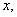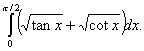Visitors Online: 77 | Monday 23rd September 2019
 CBSE Guess > Papers > Question Papers > Class XII > 2005 > Maths > Outside Delhi Set-III MATHEMATICS 2005 (Set III—Outside Delhi) Except for the following questions, all the remaining questions have been asked in Set I and Set II. SECTION - A  Q. 1. Tickets are numbered from 1 to 100. One ticket is picked up at random. Find the probability that the ticket picked up has a number which is divisible by 5 or 8. Q. 3. Find the value ofif: Q. 11. Evaluate:Q. 15. Using differentials, find the approximate value of (82) 1/4 upto 3 places of decimal. Q. 16. Using matrix method solve the following system of linear equations: SECTION - B Q. 20. Using vectors. Prove that if the diagonals of a parallelogram are equal in length, then it is a rectangle. Q. 22. A stone is dropped form a balloon that is ascending at a uniform speed of 20 m/sec. If the stone reaches the ground in 40 seconds, what was the height of the balloon when the stone was dropped? Also find the height of the balloon when the stone reaches the ground. [Use g = 10 m/sec 2 ] SECTION - C  Q. 20. For A, B and C the chances of being selected as the manager of a firm are in the ratio 4 : 1 : 2 respectively. The respectively probability for them to introduce a radical change in marketing strategy are 0.3, 0.8 and 0.5. If the change does take place, find the probability that it is due to the appointment of B or C. Q. 22. A bill for Rs. 21,900 on 19 th July, 2004 for 6 months was discounted For Rs. 21,720 at 5% per annum. Find the date on which the bill was discounted. Q. 25. A and B enter into partnership with Rs, 50,000 and Rs. 40.000 respectively. At the end of 3 months A invests Rs. 20,000 more and one month later B withdraws 1/4 th of his capital. Then centers into the businees with Rs.70,000. At the end of a year from the starting of the business, if the profit declared was Rs. 12,180, find the share of each of them in it. Maths 2005 Question Papers Class XII Delhi Outside Delhi Compartment Delhi Compartment Outside Delhi Set I Set I Set I Set I Set II Set II Set II Set II Set III Set III CBSE 2005 Question Papers Class XII